## 1.2 PROPERTIES OF INTEGERS:

Lesson Progress
0% Complete

1.2  PROPERTIES OF INTEGERS:

The sum of any two integers is always an integer. Integers are closed under addition.

For any two integers a and b, a + b is an integer.

If a and b are any two integers, then a + b = b + a

If a,b,c are any three integers, then (a + b) + c  = a + (b + c)

If ‘a’ is any integer, then a + 0 = a or 0 + a. Zero is additive identity for integers.

The sum of an integer and its opposite is 0. Thus , if ‘a’ is an integer, then a + (−a) = 0

If ‘a’ and ‘b’ are any two integers and a − b = c, then ‘c’ is also an integer.

If ‘a’ is an integer. then (a−1) is its predecessor.

If ‘a’ is any integer , then a−o =a, i.e., zero subtracted from any integer is the integer itself.

Exercise 1.2

1. Write down a pair of integers whose:
2. a) Sum is – 7         b) Difference is – 10          c) Sum is 0

Solution:  a) We can have (– 3) + (– 4) =– 7
The required pair of integers is – 3 and – 4

b) We can have – 16 – (– 6) = – 16 + 6 =  – 10
The required pair of integers is – 16 and – 6

c) We can have – 8 + 8 = 0
The required pair of integers is – 8 and 8

2.a) Write a pair of negative integers whose difference gives 8                                               b) Write a negative integer and a positive integer whose sum is –5                                       c) Write a negative integer and a positive integer whose difference is – 3.

Solution: a) Since,7 –( –15) = –7 + 15 = 8

So, – 7 and 15 is a pair of negative integers such that their difference is 8.

b) Since, (6) + 1 = 5

So, 6 and 1 is a pair of integers such that their sum is5 and one of them is a negative integer.

c) Since, –9 –( – 6) = –9 + 6 = – 3

So, – 9  and – 6 are a pair of integers such that their difference is – 3                                   and one of them is positive and other is negative.

3.In a quiz, team A scored – 40, 10, 0 and team B scored 10, 0, – 40 in three successive rounds. Which team scored more? Can we say that we can add integers in any order?

Solution:-  Total score obtained by team A =  – 40 + 10 + 0

= – 40 + 10 = – 30

Total Score of team B = 10, 0, −40

Total score obtained by team B =  10 + 0 + (– 40)

=  10 + 0 – 40  = – 30

Therefore, scores of the both A team and B team are same. – 30.

Yes, we can say that we can add integers in any order.

4.Fill in the blanks to make the following statements true:

(i) (–5) + (– 8) = (– 8) + (…………)

(ii) –53 + ………… = –53

(iii) 17 + ………… = 0

(iv) [13 + (– 12)] + (…………) = 13 + [(–12) + (–7)]

(v) (– 4) + [15 + (–3)] = [– 4 + 15] +…………

Solution:-

i) Since, integers can be added in any order,                                                                                                                                  (– 5) + (– 8) = (– 8) + (– 5)

ii) If we add zero to any integer, we get the same integer.                                                                                                             – 53 + 0 = – 53

iii) We know that the sum of an integer and its additive inverse is zero.                                                                                17 + (– 17) = 0

iv) Since, the addition of integers is associative.                                                                                               For three integers, a, b, and c,   we have: ( a + b) + c = a + (b + c)                                                               Thus, [13 +  (– 12)] + (– 7)                                                                                                                                   = 13 + [(–12) + (–7)]

v) (– 4) + [15 + (–3)] = [– 4 + 15] + (–3)
( –4) + [15 –3] = [ 11] – 3
– 4 +12 = 11 – 3
8 = 8

Try these:   (page 10)

Q.1) Using number line, find:

(i)    4 x (  –8)                          (ii)   8 x (–2)                (iii) 3               (iv) 10

Solution: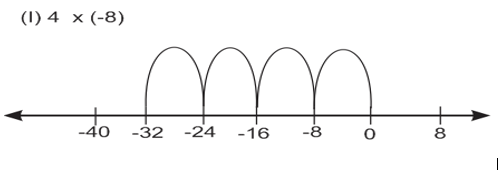From the number line, we have:

(8)  (8) (8) (8) = 32

∴ 4 x (–8) = 32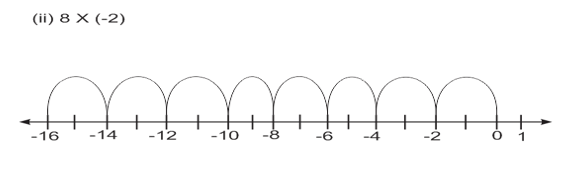From the number line, we have: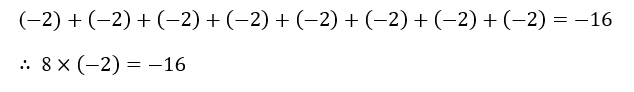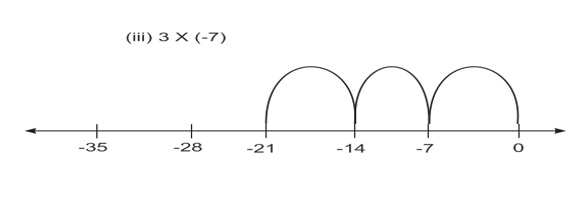From the number line, we find:

(7) + (7) + (7) = 21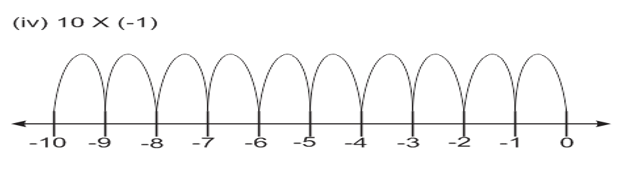From the number line, we have:

(–1)+ (–1) +(–1) +(–1)+ (–1) +(–1) +(–1)+ (–1) +(–1) +(–1) = –10

Try these:  (page 10)

i) 6  ×–19      ii) 12  ×–32     iii) 7 × –22

Solution:

i) 6 × 19=  [6  × 19] =114

ii) 12 ×–32 = [12 ×  32] = –384

iii) 7 ×–22  =  [7 ×  22] = 154

Try these: (page 11)

a) 15 × ( 16)                b) 21× ( 32)                c) (42) × 12            d) (55)× 15

Solution:

While multiplying a positive integer and a negative integer, we multiply them first and put a minus sign () before the product.

a) 15 × ( 16) = [15 × 16] = –240

b) 21  ×32 = [21 ×–32 ] = –672

c) (42)× 12 =  [42 ×12] = –504

d) (55) ×15  =    [55  ×15]= 82

Try these: (page 12)

1) Find: (–31)(–100),  (–25)(–72),  (–83)(–28)

Solution: We multiply the two negative integers and put the positive sign(+) before the product.

(31)×(100) = [  31×–100]  = + 3100

(25)×(72) = [25 ×–72] = +1800

(–83)×(–28) = [–83 ×–28] = +2324

Try these: (page 18)

i) Is 10 x [6 + (−2)] = 10 x 6 + 10 x (−2)

ii) Is (−15) x  [(−7) + (−1)] = (-15) x (-7) + (−15) x (−1)

Solution:

i) [ a x (b + c) = a x b + x c]

10 x [ 6 + (−2) ] = 10 x [ 6 − 2 ]

= 10 x 4 = 40

And, 10  x  6 + 10  x (−2) = 60 − 20 = 40.

Thus,  10 x [6 + ( –2)] = 10 x 6 +  10 ( –2)

(ii)   [ x ( b + c ) = a x b + x c]

( 15) x [ (7) + ( 1) = ( 15 ) x [  7 1 ]

= (  15 ) x ( 8 )

= ( + ) ( 15 x 8 ) = 120.

And, ( 15 ) x ( 7 ) + (   15 ) x (   1 )

= (+) ( 15 x 7 ) + ( + ) ( 15 x 1 )

= 105 + 15 = 120.

Thus, ( – 15 ) x (–7) + ( – 1 ) =(–15) x (7) + (–15) x (–1)

Try these: (Page 19)

By using distributive property find:                                                                                                                                i)  (– 49 ) x 18;        ii)  (–25) x (–31);       iii)  70 x (–19) + (–1) x 70

Solution:

i) ( – 49 ) x 18:

18 = 10 + 8

( 49 ) x 18

= ( 49 ) x [10 x 8 ]

= (  49 ) x 10 + (  49 ) x 8

Using Distributive Property we get

= 490 + (  49 ) [ 10 2 ]    because    [ 8 = 10  2 ]

= − 490 + ( 49 ) x 10 49 ) x 2

= 490 + ( 490 ) + 98

980 + 98 = 882

(ii) (  –25 ) x (–31):

31 = (  30 ) + ( 1 )

( 25 ) x (  31 )

= ( 25 ) x [ (  30 ) + (1) ]

= (25 ) x ( 30 ) + ( 25) x (1)

using Distributive Property

= + ( 25 x 30 ) + [ (25 x 1) ]

= 750 + 25 = 775

(iii) 70 x (  –19 ) + (  –1 ) x  70 :

a × b + a × c = a × [ b + c ]

70  × ( 19 ) + ( 1 ) × 70

= 70 × [ ( 19 ) + (1) ]

=  70 × [ 20 ]  = [ 70 × 20 ]   =  –1400